# How to Check Linked List is Linear or Not

## How to Check Linked List is Linear or Not in C Language

In this article, I am going to discuss How to Check Whether the Linked List is Linear or Not in C Programming Language with Examples. Please read our previous article, where we discussed How to Merge two Linked Lists in C Language with Examples.

##### How to Check Whether a Linked List is Linear or Not?

Here, we will see how to detect if our linked list is having a loop or linked list is linear.

##### What is a loop?

In the following linked list and the last node of the linked list is pointing to some other node i.e. ‘4’ in the same linked list. It’s not pointing to the first node; it is pointing to some other node. So, if the last node is pointing to some other node of the same linked list, then it is forming a loop. That means this linked list is having a loop.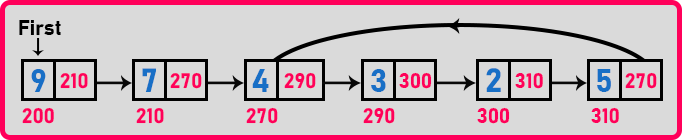In the below linked list, the last node is ending with null. So, this linked list is a linear linked list.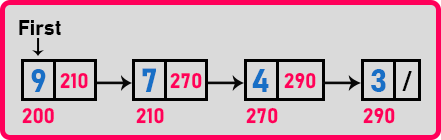So, here we have two linked lists in which the first linked list with a loop and the second linked list is linear i.e. without a loop. But the problem is if a linked list is given to us then we have to find out whether the given linked list is linear or it is having a loop. So now let us see how to detect whether the linked list is linear or not.

##### Detect whether a linked list is a linear or not:

A linked list will be linear if it is ending with null. The last node is pointing to null. So how to know whether the last node is null or not? We have to start from the first node and reach the last node and check if it is really having the value null or not. So, for that let us take a pointer ‘p’ and go on moving up to the next nodes.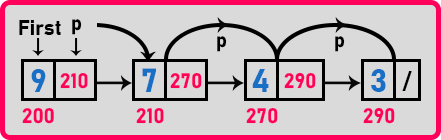And at one point ‘P’ becomes null as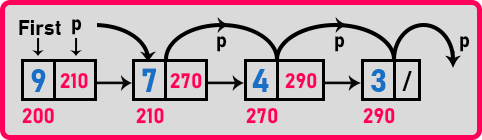So, if ‘p’ becomes null means the linked list is linear. If we are scanning through a linked list or traversing a linked list and the pointer becomes null means it is linear. Now how to know whether it has a loop or not?In the above-linked list, if we take a pointer ‘p’ and scan the linked list or traverse a single linked list then ‘p’ will move in some set of nodes only where the loop is starting. ‘p’ will start from ‘9’ and reach the end ‘5’ and again point the ‘4’ node and from here it will move again and again.

Then how we should stop ‘p’ and say that this linked list is having a loop? So, there are different solutions like storing the addresses of the nodes and if we come across the same node once again then there is a loop. Or you can store the elements if the elements are unique. If you are sure that the elements are unique then if you come over the same element once again then you can say there is a loop.

There is one more method by using two pointers. In this method, we will take two pointers on our linked list as one is ‘p’ and the other is ‘q’. Both the pointers will start from the first node.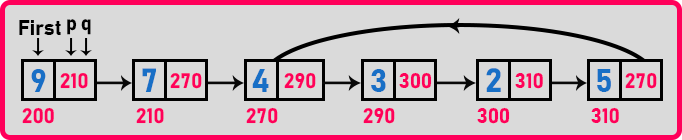‘p’ will move by one step and the ‘q’ will move by two steps. We can compare these two pointers with one example like suppose there is a race track.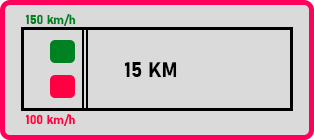So, there are two cars ‘green’ and ‘red’ on the track. The track is 15 kilometers and the green car is moving at 100 km/h speed and the red car is moving at 150 km/h speed. From the starting point once the race start then do you think they will meet again at any place? No, the green car is faster than the red car so they never meet again. Definitely green will finish the race first and then red will be reaching there. Now instead of having a straight linear track, we can have a circular track.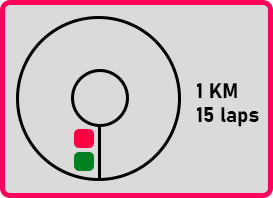Suppose this track is off 1 km long and these two cars have to take laps off of this track 15 times. So, if the red car’s speed is 100 km/h and the green car’s speed is 150 km/h. As they are going to make 15 rounds of the track then definitely, they will meet at one point. It means if one car is slow one car is fast then they never meet if the track is linear but they will meet once again if the track is circular.

So, in the same way, if the linked list is having a loop, then these two pointers will meet. If the linked list is linear, they will never meet. So let us apply the same idea to the pointers. So, move ‘p’ by one node and ‘q’ by two nodes as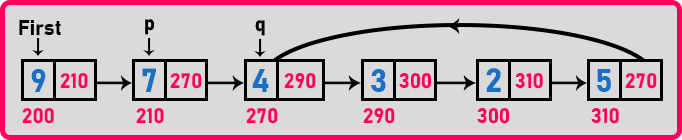Again move ‘p’ by one node and ‘q’ by two nodes,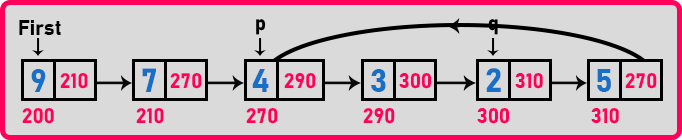Move ‘p’ by one node and ‘q’ by two nodes,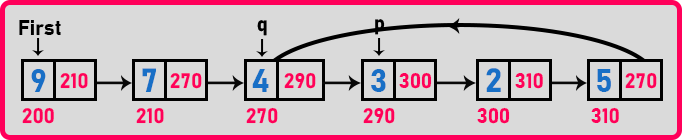Move ‘p’ by one node and ‘q’ by two nodes,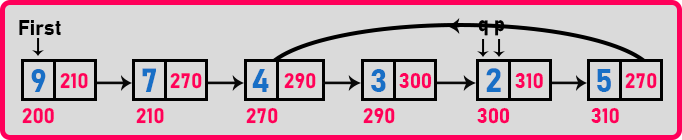At node ‘2’ they’re meeting again. After the starting point both the pointer meet once again and hence there is a loop. Let us play the same thing in the linear linked list.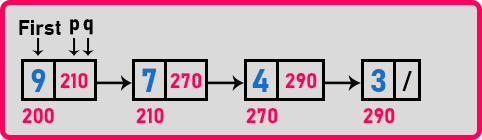Here we have ‘p’ and ‘q’ on the first node of the linked list. Now let us move ‘p’ by one and ‘q’ by two nodes,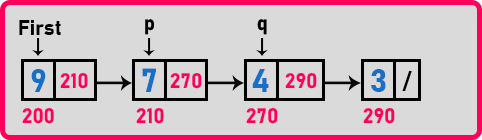Again, same steps,Here ‘q’ becomes null. So, if any of the pointers become null, we should stop saying that this linked list is linear. In a linear linked list, ‘q’ will become null or the pointer which is moving by two nodes will become null because it is moving faster.

So, we can take two-pointers and find out whether it is having a loop or it is linear. If they meet again then there is a loop. If one of the pointers becomes null then they are linear. Now let us write the function for checking whether there is a loop or not.

##### Pseudo Code for Checking if the Linked List is Linear or Not:

int IsLoop(struct Node *f){
struct Node *p, *q;
p = q = f;
do{
p = p->next;
q = q->next;
q = q ? q->next: q;
} while(p && q && p != q);
return p == q? 1:0;
}

Now let us write a complete program for checking loop in a linked list.

##### Program for Checking if the Linked List is Linear or Not in C Language:
```#include <stdio.h>
#include <stdlib.h>

struct Node
{
int data;
struct Node *next;
} *temp = NULL, *first = NULL;

struct Node* Create (int A[], int n)
{
int i;
struct Node *t, *last;
temp = (struct Node *) malloc(sizeof(struct Node));
temp->data = A;
temp->next = NULL;
last = temp;

for (i = 1; i < n; i++)
{
t = (struct Node *) malloc (sizeof (struct Node));
t->data = A[i];
t->next = NULL;
last->next = t;
last = t;
}
return temp;
}

int IsLoop(struct Node *f)
{
struct Node *p, *q;
p = q = f;
do
{
p = p->next;
q = q->next;
q = q ? q->next : q;
}
while (p && q && p != q);
return p == q ? 1 : 0;
}

int main()
{
struct Node *t1, *t2;
int A[] = { 3, 4, 7, 9 };
first = Create(A, 4);

t1 = first->next->next;
t2 = first->next->next->next;
t2->next = t1;

if (IsLoop(first))